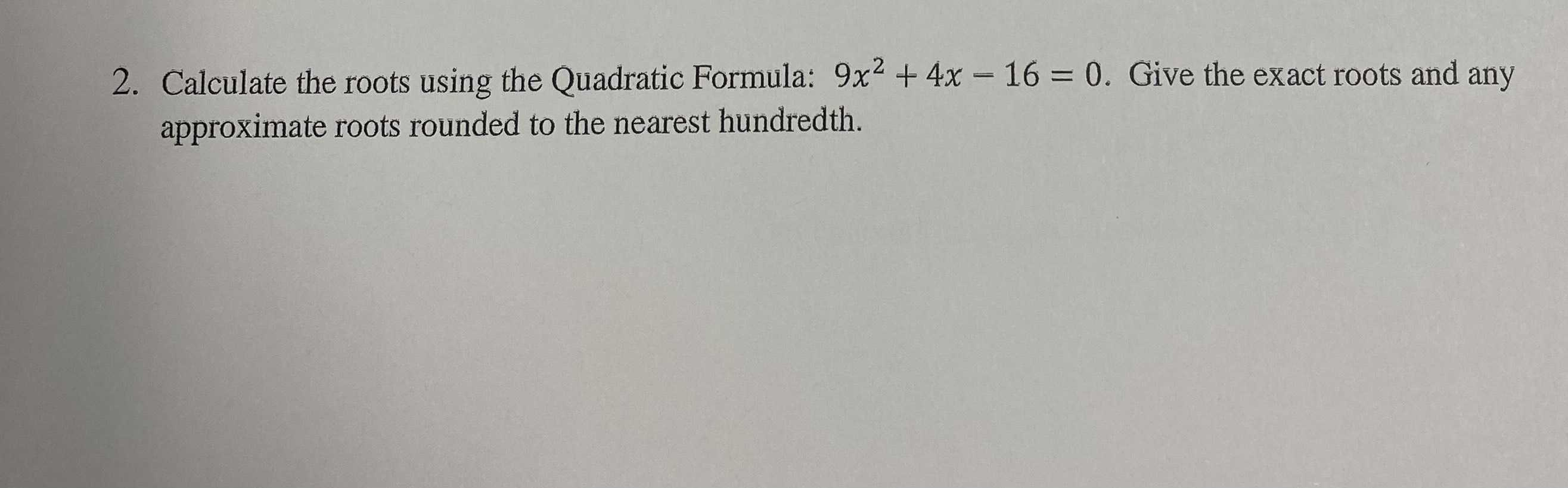### ¿Todavía tienes preguntas de matemáticas?

Pregunte a nuestros tutores expertos
Algebra
Pregunta2. Calculate the roots using the Quadratic Formula: $$9 x ^ { 2 } + 4 x - 16 = 0$$ . Give the exact roots and any approximate roots rounded to the nearest hundredth.